# Fuzzy vs. Nonfuzzy Logic

In this example, to illustrate the value of fuzzy logic, you examine both linear and fuzzy approaches to determining the correct amount to tip a waitperson at a restaurant. First, you use a conventional nonfuzzy approach that defines piecewise-linear relations between the inputs (service and food quality) and the output (tip percentage). Then, you implement a fuzzy approach using linguistic variables and if-then rules.

### Basic Tipping Problem

The basic tipping problem in this examples is as follows—given a number from 0 through 10 that represents the quality of service at a restaurant, where 10 is excellent, and another number from 0 through 10 that represents the quality of the food, where 10 is delicious, what should the tip be?

Tipping behavior varies depending on local traditions and personal preferences. In this example, the problem is based on tipping as it is typically practiced in the United States. An average tip for a meal in the US is 15%. A generous tip could be as high as 25% and a cheap tip could be 5%.

The actual amount of the tip can vary depending on the quality of the service and food.

### Nonfuzzy Approach

As a starting point, consider the simplest possible relationship, that is, the tip always equals 15% of the total bill.

```S = 0:.5:10; tip = 0.15*ones(size(S)); plot(S,tip) xlabel('Service') ylabel('Tip') ylim([0.05 0.25])```This relationship does not account for the quality of the service, so you must add a service term $\mathit{S}$ to the equation for tip $\mathit{T}$. Since service is rated on a scale from 0 through 10, increase the tip linearly from 5% if the service is bad to 25% if the service is excellent.

`$\mathit{T}=0.05+\frac{0.2\cdot \mathit{S}}{10}$`

Plot the resulting relation.

```tip = (.20/10)*S + 0.05; plot(S,tip) xlabel('Service') ylabel('Tip') ylim([0.05 0.25])```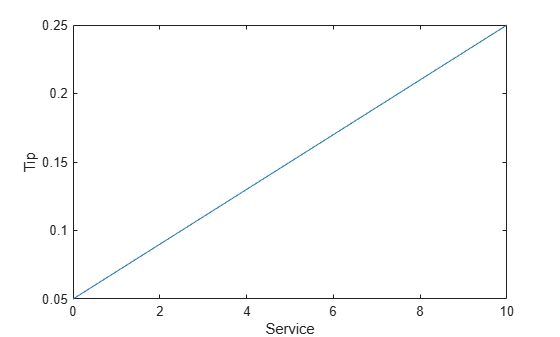To account for the food quality, add a food-quality term $\mathit{F}$ to the tip formula. Here, the service and food are rated as equally important in the tip calculation.

`$\mathit{T}=0.05+\frac{0.2\cdot \left(\mathit{S}+\mathit{F}\right)}{20}$`

Plot the resulting relation.

```food = 0:.5:10; [F,S] = meshgrid(food,S); tip = (0.20/20).*(S+F) + 0.05; surf(S,F,tip) xlabel('Service') ylabel('Food') zlabel('Tip')```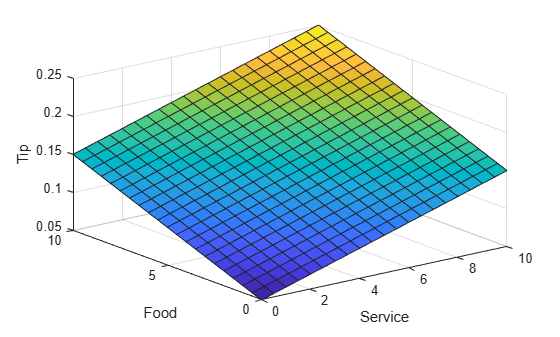In this case, the results look satisfactory. However, suppose that you want the service to be a more important factor than the food quality. To do so, you can add a service ratio $\mathit{R}$ to the formula.

`$\mathit{T}=0.05+\frac{0.2\cdot \left(\mathit{R}\cdot \mathit{S}+\left(1-\mathit{R}\right)\cdot \mathit{F}\right)}{10}$`

Specify that service accounts for 80% of the overall tipping grade and the food makes up the other 20%.

```R = 0.8; tip = (0.2/10)*(R*S + (1-R)*F) + 0.05; surf(S,F,tip) xlabel('Service') ylabel('Food') zlabel('Tip')```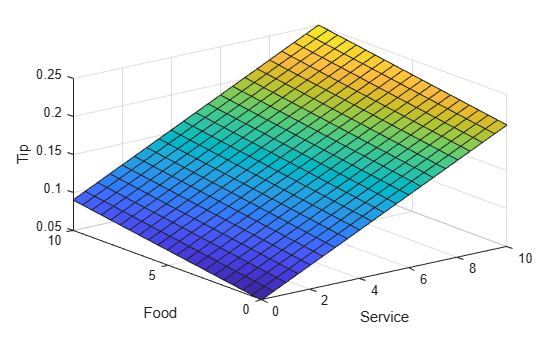Suppose further that you want a flatter response in the middle; that is, you want to give a 15% tip in general, but you also want to specify a variation when the service is exceptionally good or bad. In this case, the previous linear mappings are not sufficient. Instead, you can create a piecewise-linear construction. Returning to the service-only calculation, create a conditional tip assignment using logical indexing.

```tip = zeros(size(S)); tip(S<3) = (0.10/3)*S(S<3) + 0.05; tip(S>=3 & S<7) = 0.15; tip(S>=7 & S<=10) = ... (0.10/3)*(S(S>=7 & S<=10)-7) + 0.15; plot(S,tip) xlabel('Service') ylabel('Tip') ylim([0.05 0.25])```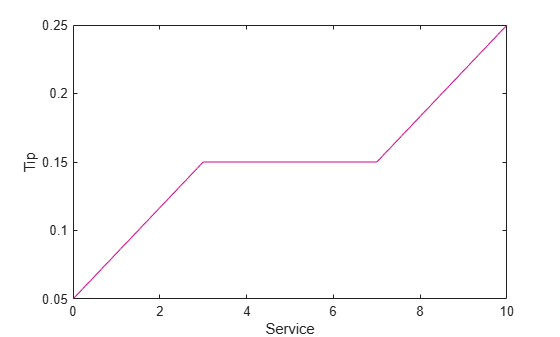Then, add a linear dependence on food service to the piecewise-linear service formula.

```R = 0.8; tip = zeros(size(S)); tip(S<3) = ((0.10/3)*S(S<3)+0.05)*R + ... (1-R)*(0.20/10*F(S<3)+0.05); tip(S>=3 & S<7) = (0.15)*R + ... (1-R)*(0.20/10*F(S>=3 & S<7)+0.05); tip(S>=7 & S<=10) = ((0.10/3)*(S(S>=7 & S<=10)-7)+0.15)*R + ... (1-R)*(0.20/10*F(S>=7 & S<=10)+0.05); surf(S,F,tip) xlabel('Service') ylabel('Food') zlabel('Tip')```The plot looks good, but the calculation is complicated. It is not apparent how the algorithm works to someone who did not see the original design process.

### Fuzzy-Logic Approach

To solve this problem using fuzzy logic, first capture the essentials of the desired tipping behavior, leaving aside all the factors that could be arbitrary. If you make a list of what really matters in this problem, you might create the following rule descriptions for tipping based on service quality.

• If service is poor, then tip is cheap.

• If service is good, then tip is average.

• If service is excellent, then tip is generous.

Similarly, for tipping based on food quality, you might create the following rules.

• If food is rancid, then tip is cheap.

• If food is delicious, then tip is generous.

Then, combine these rules into three compound if-then rules.

• If service is poor or the food is rancid, then tip is cheap.

• If service is good, then tip is average.

• If service is excellent or food is delicious, then tip is generous.

The rules use linguistic terms, such as cheap and delicious, to define the levels of service, food quality, and tip.

To implement the fuzzy logic-based solution, you create a fuzzy inference system (FIS) that contains the if-then rule base and defines the linguistic terms used in the rules.

Load a fuzzy inference system (FIS) that implements the rule-based solution.

`fis = readfis('tipper');`

View the rules for the FIS, which match the rules defined previously.

`fis.Rules`
```ans = 1x3 fisrule array with properties: Description Antecedent Consequent Weight Connection Details: Description __________________________________________________________ 1 "service==poor | food==rancid => tip=cheap (1)" 2 "service==good => tip=average (1)" 3 "service==excellent | food==delicious => tip=generous (1)" ```

This FIS has two inputs (service and food quality) and one output (tip percentage). Each input and output variable contains membership functions that define the linguistic terms used in the if-then rules.

For example, the following membership functions represent the tip percentage.

`plotmf(fis,"output",1)`View the input-output relation defined by this FIS.

`gensurf(fis)`This fuzzy system is based on a set of common sense rules that are easily understandable by someone who did not create the system.

To change the behavior of this system for different regions or personal preferences, you can add or modify rules, variable ranges, and linguistic term definitions. For example, to adjust the average, minimum, and maximum tip values, you can change the range of the output variable and modify the membership functions accordingly.

As an example, shift the tip range up by five percent.

```fis.Outputs(1).Range = fis.Outputs(1).Range + 5; fis.Outputs(1).MembershipFunctions(1).Parameters = ... fis.Outputs(1).MembershipFunctions(1).Parameters + 5; fis.Outputs(1).MembershipFunctions(2).Parameters = ... fis.Outputs(1).MembershipFunctions(2).Parameters + 5; fis.Outputs(1).MembershipFunctions(3).Parameters = ... fis.Outputs(1).MembershipFunctions(3).Parameters + 5; plotmf(fis,"output",1)```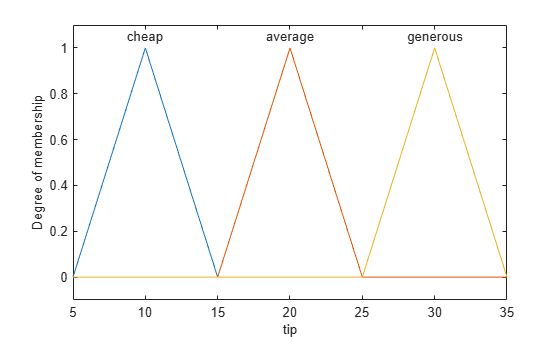The tipping logic of the FIS, as defined by the if-then rule base, remains the same. However the definition of what the different tip levels mean has changed.

For more complicated changes, you can modify the rule base by modifying existing rules or adding and removing rules.